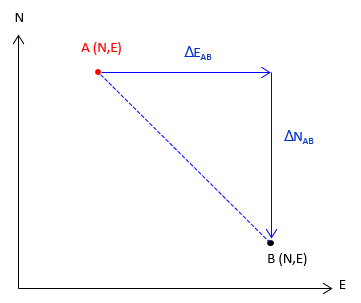### 2. Positions

#### a. Absolute: Coordinates

The absolute position of a point is expressed as coordinates which are perpendicular distances from the reference axes. In surveying and most mapping, the reference system uses North and East axes so position is expressed as (North, East) (aka, Northing and Easting), Figure A-3. Other applications, notably math, use X and Y axes; Y coinciding with the meridian. Positions are expressed as (X, Y), Figure A-4.Figure A-3 (North, East) Coordinates Figure A-4 (X, Y) Coordinates

Caution must be exercised when using coordinates provided by others. Are (2000 ft,1000 ft) North-East or X-Y coordinates? A common error when importing coordinates in software is reversing their order: this flips and rotates the positions. This effect is demonstrated in Figures A-5 and A-6. The former uses coordinates in (N, E) order while the latter in (X, Y).Figure A-5 Data Correctly Imported as (N, E) Coordinates Figure A-5 Data Incorrectly Imported as (X, Y) Coordinates

We will use the North-East coordinate form unless otherwise specified.

#### b. Relative

A relative position is where one point is with respect to another. This can be expressed as coordinate differences, Figure A-6, or as distance and direction, Figure A-7.Figure A-6 Coordinate Differences Figure A-7 Distance and Direction